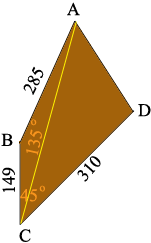SEARCH HOMEMath Central Quandaries & QueriesQuestion from Emran, a student: I have a irregular polygon. I know 3 of the 4 sides, and 2 of the angles. A-B is 285, B-C is 149, and C-D is 310. Angle B is 135 degrees. and Angle C is 45 degrees. Is there a formula to solve for the final side? Thanks.Hi Emran,

I drew a diagram (not to scale) of what you describe.I added one line segment from A to C. This partitions the polygon into two triangles, ABC and ACD. Application of the laws of sines and cosines to these two triangles will allow you to find what you need.

Try it and if you need more assistance write back and tell us what you did,
PennyMath Central is supported by the University of Regina and The Pacific Institute for the Mathematical Sciences.